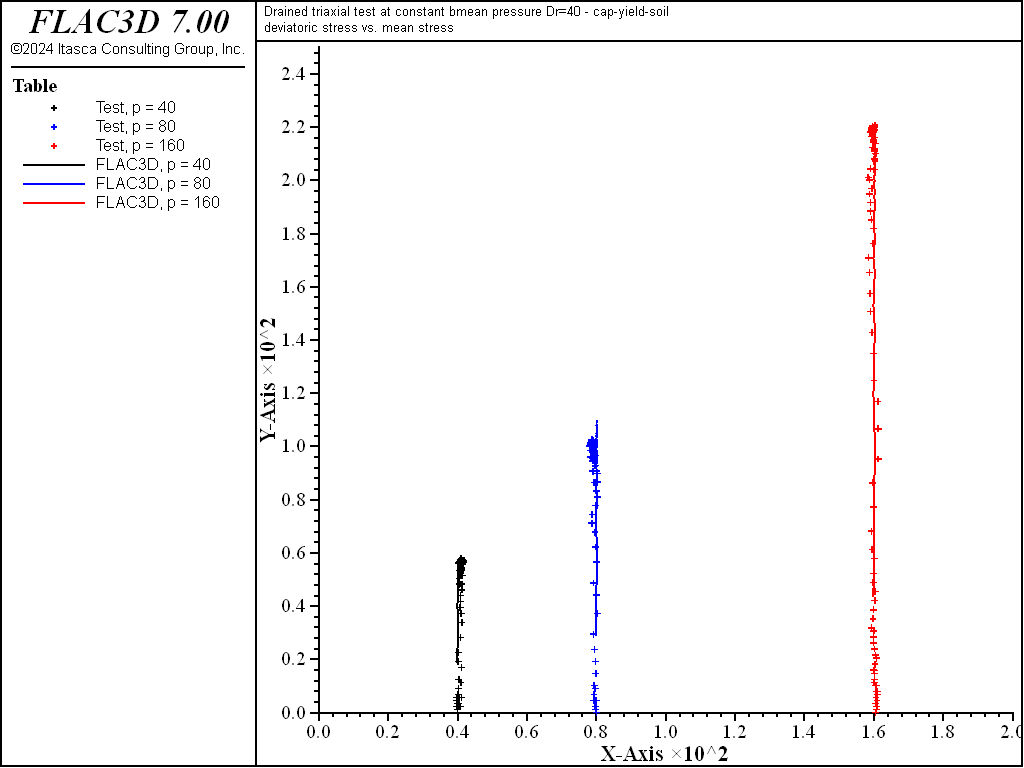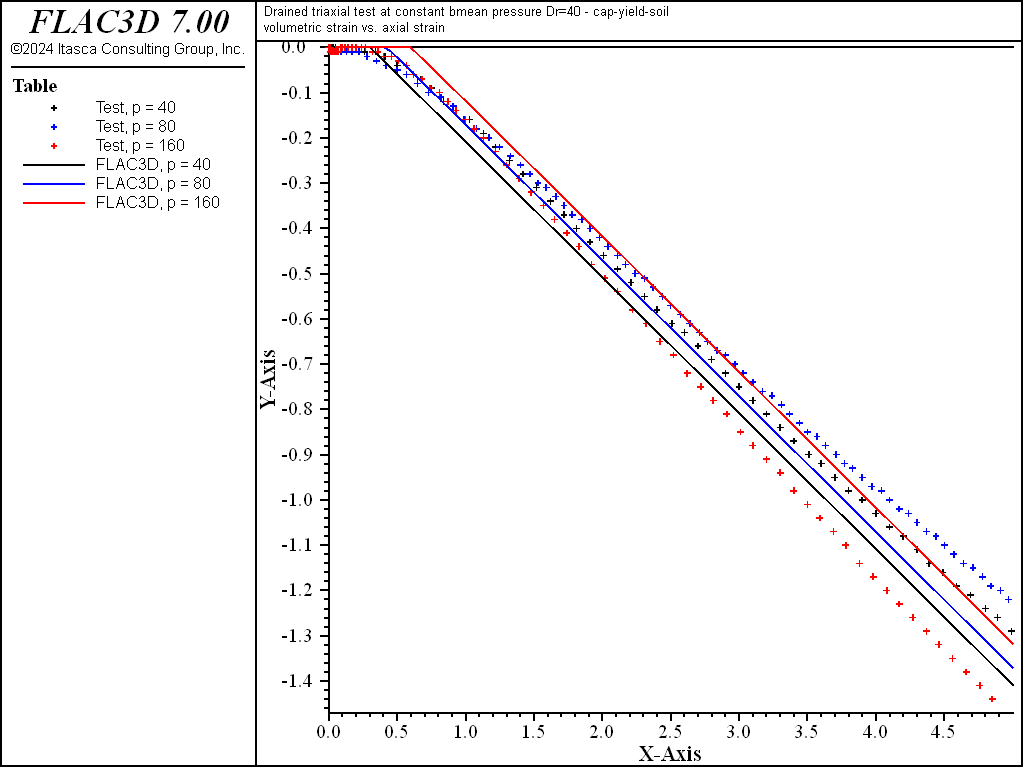FLAC3D Theory and Background • Constitutive Models

# Drained Triaxial Compression Test with Simplified Cap-Yield (CHSoil) Model

Note

To view this project in FLAC3D, use the menu command Help ► Examples…. Choose “ConstitutiveModels/ DrainedTriaxialCHSoil” and select “DrainedTriaxialCHSoil.prj” to load. The project’s main data file is shown at the end of this example.

Triaxial experiments are conducted numerically using the CHSoil model at the three levels of constant mean stress, 40, 80, and 160 kPa, for the $$D_r$$ = 40% sand. A servo-control is applied to maintain the mean stress constant during the numerical experiments.

The estimates for model properties are used to conduct the numerical tests, and the test results are compared to the available laboratory data (imported into FLAC3D in tables). The properties are adjusted (see the section on calibration), and the numerical experiment is repeated until a satisfactory curve fitting is obtained.

The results of the curve fitting experiment are listed in Table 1.

 $$D_r$$ $$E_{ref}$$ $$\nu$$ $$\phi_f$$ $$\psi_f$$ $$\phi_{cv}$$ $$m$$ $$n$$ 40% 1800 0.35 34° 7.5° 28° 0.5 0.5

A comparison between numerical predictions using the calibrated properties and laboratory results is shown in Figure 1 through Figure 3. Note that the soil-mechanics convention for positive stress/strain is adopted in these plots. (Dilation is negative.) The comparison is quite reasonable.Figure 1: Deviator stress versus mean stress for $$D_r$$ = 40%—comparison between laboratory (line) and numerical (cross) results.Figure 2: Deviatoric stress versus axial strain (in %) for $$D_r$$ = 40%—comparison between laboratory (line) and numerical (cross) results.Figure 3: Volumetric strain (in %) versus axial strain (in %) for $$D_r$$ = 40%—comparison between laboratory (line) and numerical (cross) results.

Data File

DrainedTriaxialCHSoil.dat

model new
model title ...
'Drained triaxial test at constant bmean pressure Dr=40 - cap-yield-soil'
model large-strain off
fish automatic-create off
;
zone create brick size 1 1 5
zone cmodel assign cap-yield-simplified
zone property young-reference=1800 poisson=0.35 pressure-reference=100.0
zone property failure-ratio=0.99 friction=34.0 exponent-bulk=0.5 ...
exponent-shear=0.5
zone property density=1000. cohesion=0.0 dilation-mobilized=0.0 ...
friction-mobilized=0.0
zone property flag-dilation=2 friction-critical=28.0 dilation=7.5
zone property pressure-initial= 40.0 range id = 1
zone property pressure-initial= 80.0 range id = 3
zone property pressure-initial=160.0 range id = 5
;
zone cmodel assign null range id-list = 2, 4
;
zone initialize stress xx  -40.0 yy  -40.0 zz  -40.0 range id = 1
zone initialize stress xx  -80.0 yy  -80.0 zz  -80.0 range id = 3
zone initialize stress xx -160.0 yy -160.0 zz -160.0 range id = 5
;
zone gridpoint fix velocity
zone gridpoint initialize velocity-x 0.25e-6 range position-x 1.0
zone gridpoint initialize velocity-y 0.25e-6 range position-y 1.0
zone gridpoint initialize velocity-z -0.5e-6 range union position-z 1.0 ...
position-z 3.0 position-z 5.0
;
[global z1 = zone.near(0.5,0.5,0.5)]
[global z3 = zone.near(0.5,0.5,2.5)]
[global z5 = zone.near(0.5,0.5,4.5)]
;
program call 'servo'
;
fish history q1
fish history p1
fish history eps_v1 ; vol. strain (%) dilation positive
fish history eps_a1 ; axial strain (%)
fish history q2
fish history p2
fish history eps_v2 ; vol. strain (%) dilation positive
fish history eps_a2 ; axial strain (%)
fish history q3
fish history p3
fish history eps_v3 ; vol. strain (%) dilation positive
fish history eps_a3 ; axial strain (%)
history interval 1000
model step 100000
model save 'chsoil-dtriax1'